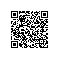# MobileNet教程(2)：用TensorFlow搭建安卓手机上的图像分类App

MobileNet是为移动端量身打造的，因此这次我们准备把之前做的辨别道路的模型应用到一个Android App中，看看它在移动设备上效果如何。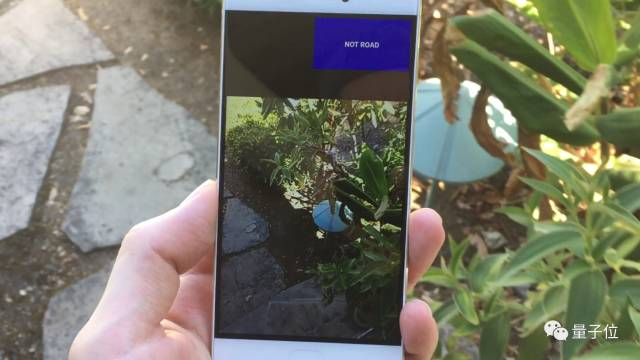# 目标和计划

• 在很小的特定数据上重新训练MobileNet；
• 模型在hold out测试集（即模型训练前为测试留出的样本）上达到95%的准确率；
• 程序运行时对300美元以下价位手机CPU的占用要小于5%。

1. 生成一个新的训练数据集；
2. 训练多个MobileNet结构，从而寻找所能够达到准确率目标（95%）的最小型网络；
3. 与在Android上运行的Inception V3做对比；
4. 将TensorFlow上Android example App中的模型替换为我们的MobileNet；
5. 大量的测试；
6. 进行调试，从而将CPU的占用调到5%以下。

# 建立数据集###### △ 一些“非道路”的示例图片# 用特定数据集训练MobileNet

python tensorflow/examples/image_retraining/retrain.py \
--learning_rate=0.0005 \
--testing_percentage=15 \
--validation_percentage=15 \
--train_batch_size=32 \
--validation_batch_size=-1 \
--flip_left_right True \
--random_scale=30 \
--random_brightness=30 \
--eval_step_interval=100 \
--how_many_training_steps=1000 \
--architecture mobilenet_1.0_128_quantized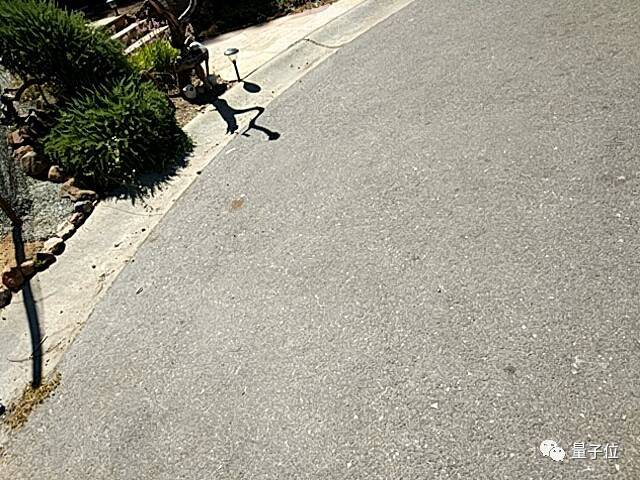###### △ 被错认为道路的非道路图片，我不得不说这种失误是可以接受的，这显然是路，但不是我们要的类型 。###### △ 被错认为非道路的道路图片，我认为这是因为在训练集中没有出现桥架在道路上的图片，更多的训练数据能解决这个问题。

python tensorflow/examples/label_image/label_image.py \
--graph=/tmp/output_graph.pb \
--labels=/tmp/output_labels.txt \
--input_layer=input \
--output_layer=final_result \
--input_mean=128 \
--input_std=128 \
--input_width=128 \
--input_height=128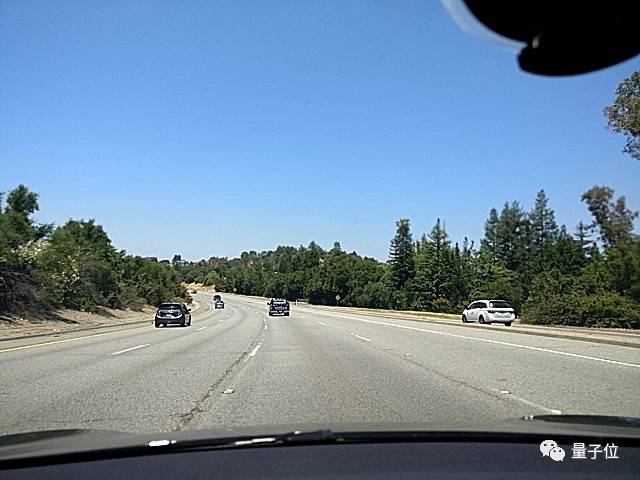# 把MobileNet应用到Android App中

## 1. 建立项目

git clone https://github.com/tensorflow/tensorflow.git --depth 1

## 2. 评测搭载了Inception的App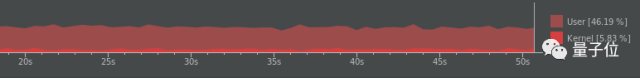###### △ 搭载Inception V3的App在4fps速度下运行时的CPU占用情况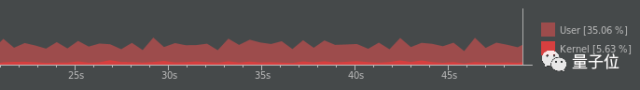## 3. 换成MobileNet

tensorflow/examples/android/src/org/tensorflow/demo/ClassifierActivity.java

private static final int INPUT_SIZE = 224;
private static final int IMAGE_MEAN = 117;
private static final float IMAGE_STD = 1;
private static final String INPUT_NAME = "input";
private static final String OUTPUT_NAME = "output";

private static final String MODEL_FILE = "file:///android_asset/tensorflow_inception_graph.pb";
private static final String LABEL_FILE =    "file:///android_asset/imagenet_comp_graph_label_strings.txt";

private static final int INPUT_SIZE = 128;
private static final int IMAGE_MEAN = 128;
private static final float IMAGE_STD = 128;
private static final String INPUT_NAME = "input";
private static final String OUTPUT_NAME = "final_result";

private static final String MODEL_FILE = "file:///android_asset/output_graph.pb";
private static final String LABEL_FILE =    "file:///android_asset/output_labels.txt";

# 结果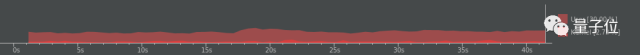###### △ 搭载MobileNet的App在18fps速度下运行时的CPU占用情况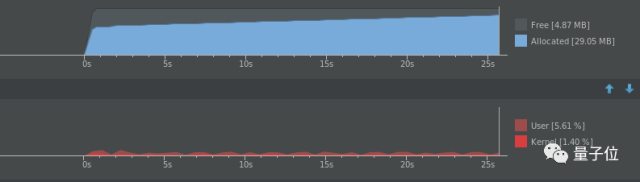# 相关链接

https://hackernoon.com/building-an-insanely-fast-image-classifier-on-android-with-mobilenets-in-tensorflow-dc3e0c4410d4

—— ——